# Algebra II : Frequency Distributions

## Example Questions

### Example Question #1 : Frequency Distributions

The number of runs scored per game by a little league baseball team is represented by the following frequency distribution:

 Number of Runs Scored Frequency 0 5 1 2 2 7 3 9 4 3 5 0 6 4 7 2 8 2 9 1

Using this frequency table, select the correct answer of mean runs scored by the little league team (rounded to the nearest hundredth).

Possible Answers: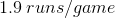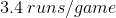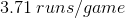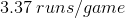There's not enough information present to determine the mean.

Correct answer:Explanation:
 Number of Runs Scored Frequency 0 5 1 2 2 7 3 9 4 3 5 0 6 4 7 2 8 2 9 1

To determine the mean, first the data set should be re-written in sequential order: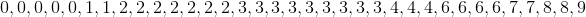The formula to determine mean is: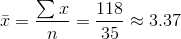The mean is approximately 3.37 runs scored per game.

### Example Question #2 : Frequency Distributions

Thousands of people in the United States were surveyed about their grandparents. This frequency table shows their answer to the question "how many of your grandparents were born outside of the US?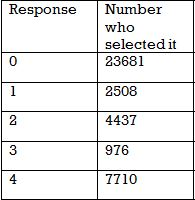Of the people who had at least one grandparent born outisde of the US, what percent had exactly 3 non-US born grandparents?

Possible Answers: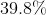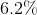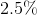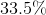Correct answer:Explanation:

Adding up the total number of grandparents that were born outside the U.S. is our first step in solving this problem.

There are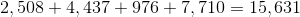people who said they had at least 1 grandparent born outside of the US. Of those, only 976 had exactly 3.

So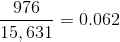oris the answer.

### Example Question #3 : Frequency Distributions

Thousands of people in the United States were surveyed about their grandparents. This frequency table shows their answer to the question "how many of your grandparents were born outside of the US?"Is this data normally distributed?

Possible Answers:

Yes

Partly

No

Need more information

Correct answer:

No

Explanation:

No - normally distributed data has a low frequency of responses at the high and low ends. Also, the majority of the data is in the middle.

In this data set, the majority of people said "0," and the fewest people said "3" which is near the middle of the data. If this data was normally distributed we would have a higher frequency between 1 and 3 and much lower values for 0 and 4.

### Example Question #4 : Frequency Distributions

What is the correct frequency distribution for this data set?

1   3   5   2   5   2   4   1   5
2   4   1   2   3   5   5   2   3
3   1   4   4   6   2   3   2   4

Possible Answers: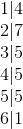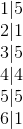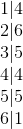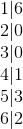Correct answer:Explanation:

Count the number of times each number appears in the data set.

The number 1 appears 4 times, so the first line is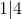.

Then number 2 appears 7 times, so the next line is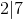.

Continuing in this fashion we see that the numbers 3, 4, and 5 appears 5 times and 6 appears once.

Therefore combining these together we get the following answer:### Example Question #5 : Frequency Distributions

What is the mean of the data in this frequency table?

#   Frequency
1    3
2    4
3    0
4    2

Possible Answers: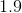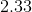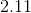Correct answer:Explanation:

The frequency table represents the data set:

1, 1, 1, 2, 2, 2, 2, 4, 4.

The sum is 19 and there are 9 data points, so the mean is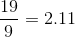.

### Example Question #6 : Frequency Distributions

What is the median of the data in this frequency table?

#   Frequency
1    3
2    4
3    0
4    2

Possible Answers:Correct answer:Explanation:

The frequency table represents the data set:

1, 1, 1, 2, 2, 2, 2, 4, 4.

The median is 2 because 4 data points appear before and after the center 2.

### Example Question #7 : Frequency Distributions

Which frequency table has a median of 5? (The first column represents the data points, the second column the frequency)

Possible Answers: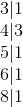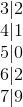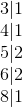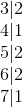Correct answer:Explanation:

The correct frequency table represents the data set 3, 4, 5, 5, 6, 6, 8.

Since there are 7 data points our median will be the 4th spot which makes the value 5. It occures at the 4th spot because at that spot half the data points are below it while the other half are above it.

The center number of that data set is 5 as required.

### All Algebra II Resources